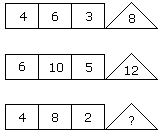# Verbal Reasoning - Character Puzzles - Discussion

### Discussion :: Character Puzzles - Character Puzzles 1 (Q.No.18)

18.

Which one will replace the question mark ?[A]. 8 [B]. 12 [C]. 16 [D]. 20

Explanation:

(4 x 6) % 3 = 8

(6 x 10) % 5 = 12

(4 x 8) % 2 = 16.

 Darkprince said: (Sep 3, 2011) I first thought of like this :- 6 + 2 x ( 4 -3) =8 10 + 2 x (6-5) =12

 Giridhar said: (May 9, 2016) It's, (4 * 6)/3 = 8. (6 * 10)/5 = 12. (8 * 4)/2 = 16.

 Biniyam said: (Jul 28, 2016) What if. 8 = (4 * 6) - (6 * 3) + 2. 12 = (6 * 10) - (10 * 5) + 2. (4 * 8) - (8 * 2) + 2 = 18. Am I right.

 Subhashree said: (Sep 25, 2016) Why %2?

 Sagar said: (Oct 9, 2016) I thought that the difference is 4.

 Herb said: (May 1, 2017) What is the use of % here? Please explain in detail.

 Parameswarawarman said: (Jun 11, 2017) 4*6=24 (4 6 3 =8) 24/3 so 8. 6*10=60 (6 10 5 =12) 60/5 so 12. Same way 4 * 8 = 32 (4 8 2=?) 32/2 = 16.

 Anonymous said: (Jun 19, 2021) It can also be (1st + 2nd) - (3rd+1). Whether my logic is right?